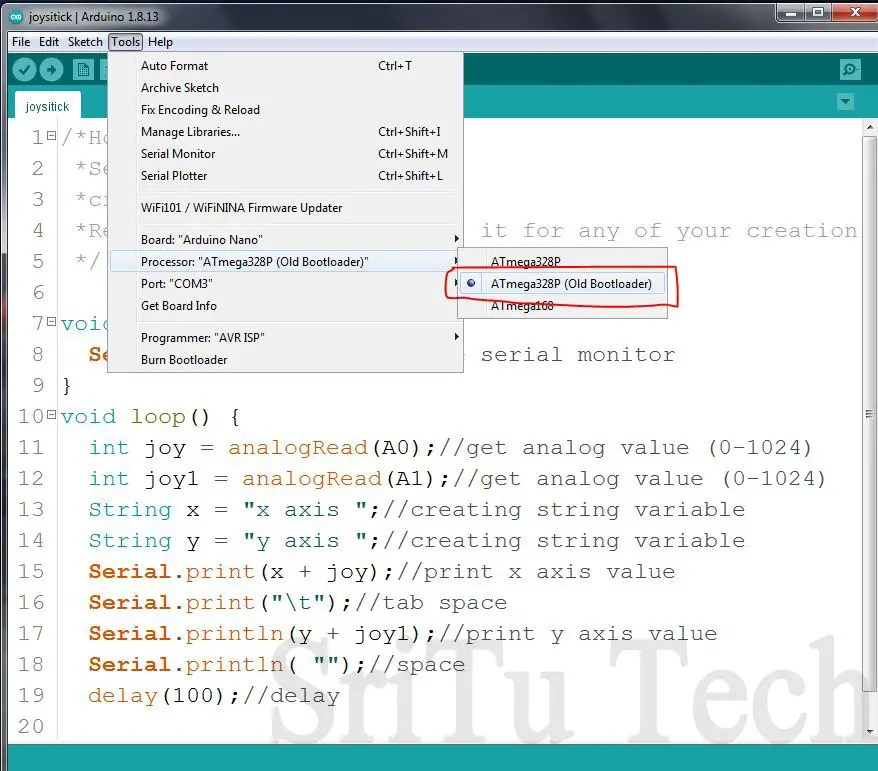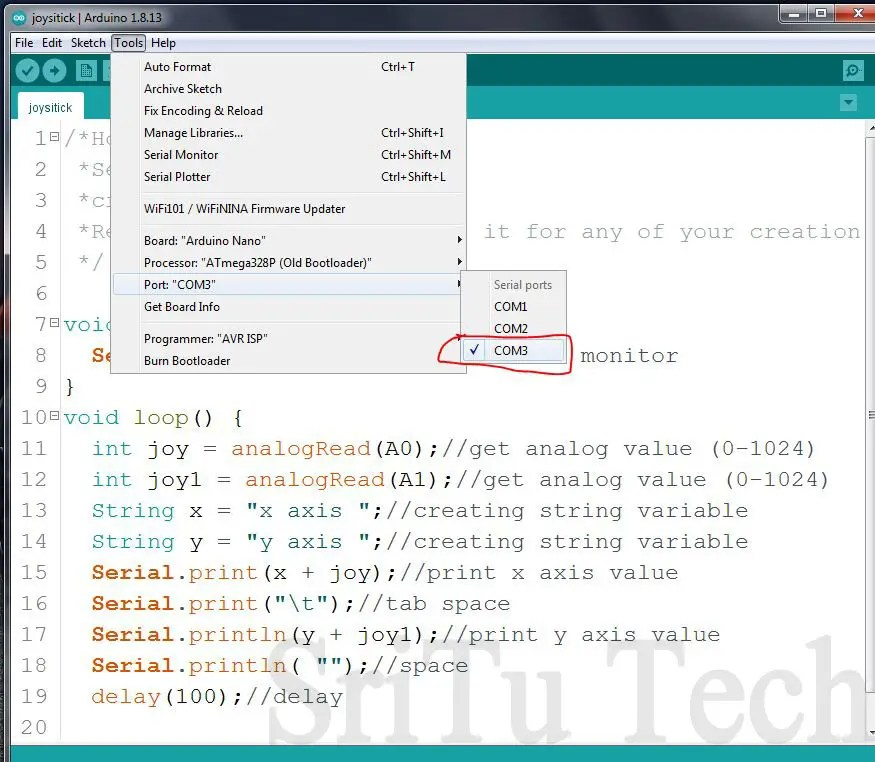# Joystick module with Arduino.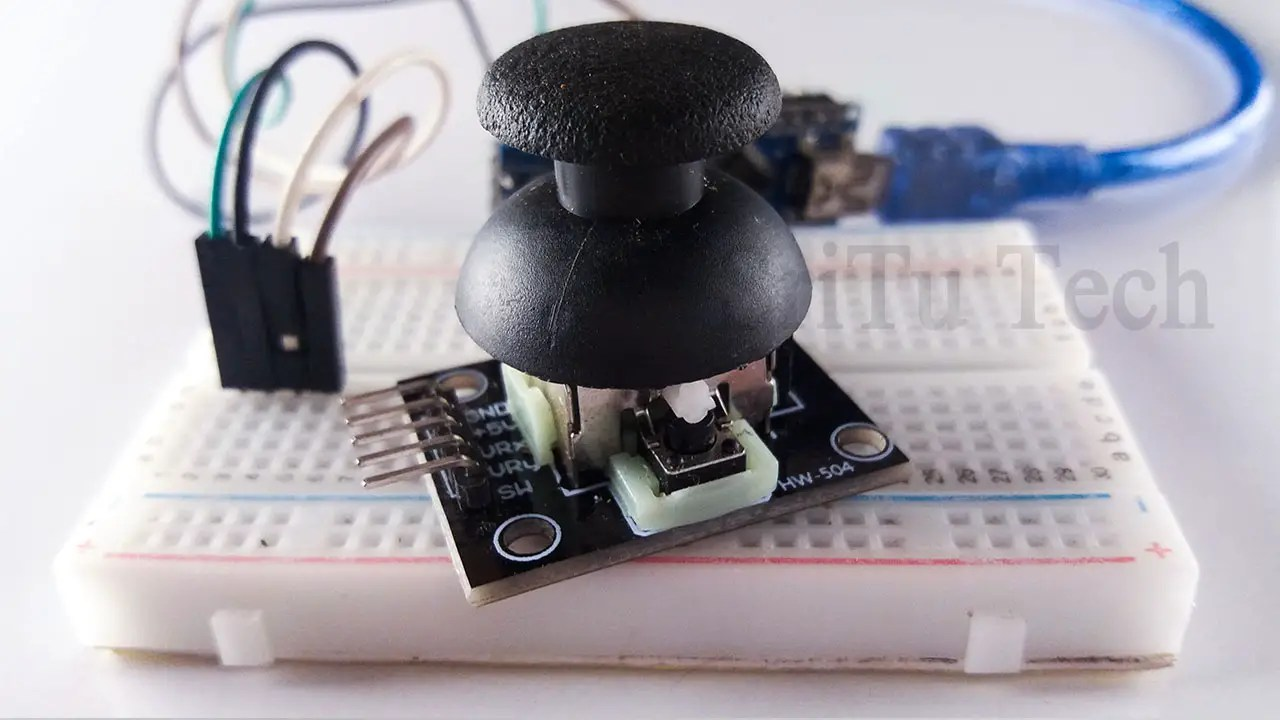Hello guys, Welcome back to my blog. We have already described the types of data required for Arduino encoding in the previous post. We create programs using that knowledge. Okay, today we are going to do a small project and learn Arduino. For that, I have used digital read, analog read and analog write. The following are articles describing these.
Analog write.

Okay, let’s go to the post today. We have mainly used the joystick module for this project. We have seen this component in game consoles and in remote controls. They cannot be customized. But with the module we are talking about today, we can do whatever we want through the Arduino board. Let’s do this project using simple code. The following is this module.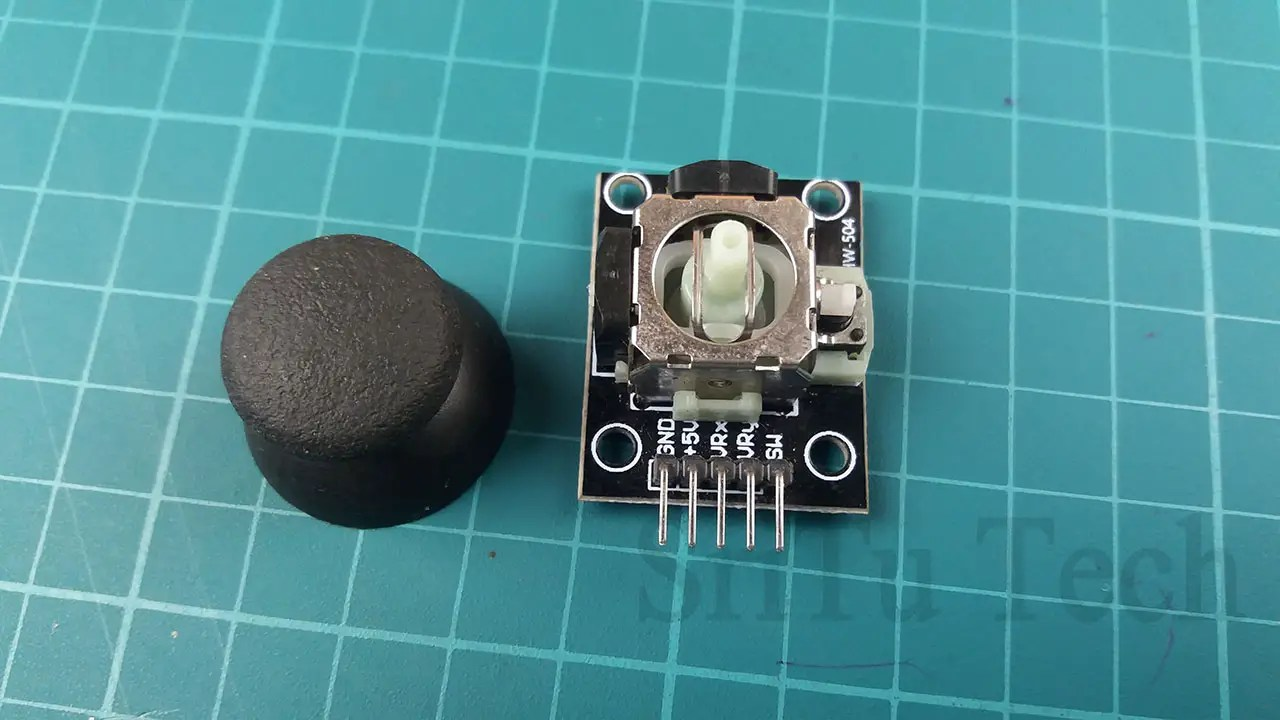Using this component we can create remote controls and game consoles. We will talk about these projects in the next articles. This joystick module has five pins. These are GND, + 5v, VRx, VRy, and SW. It uses GND and + 5v pins to power this component. Analog values ​​can be obtained via VRx and VRy pins by moving this module to both the X and Y axes. A digital value can be obtained from the SW pin. To do this, the module must be pushed down. Let’s do this project practically, using a servo motor and an LED bulb. The required components are as follows.

### Components

Ok, let’s do this project step by step.

Step 1
Let’s Identify these components.
Arduino Uno board.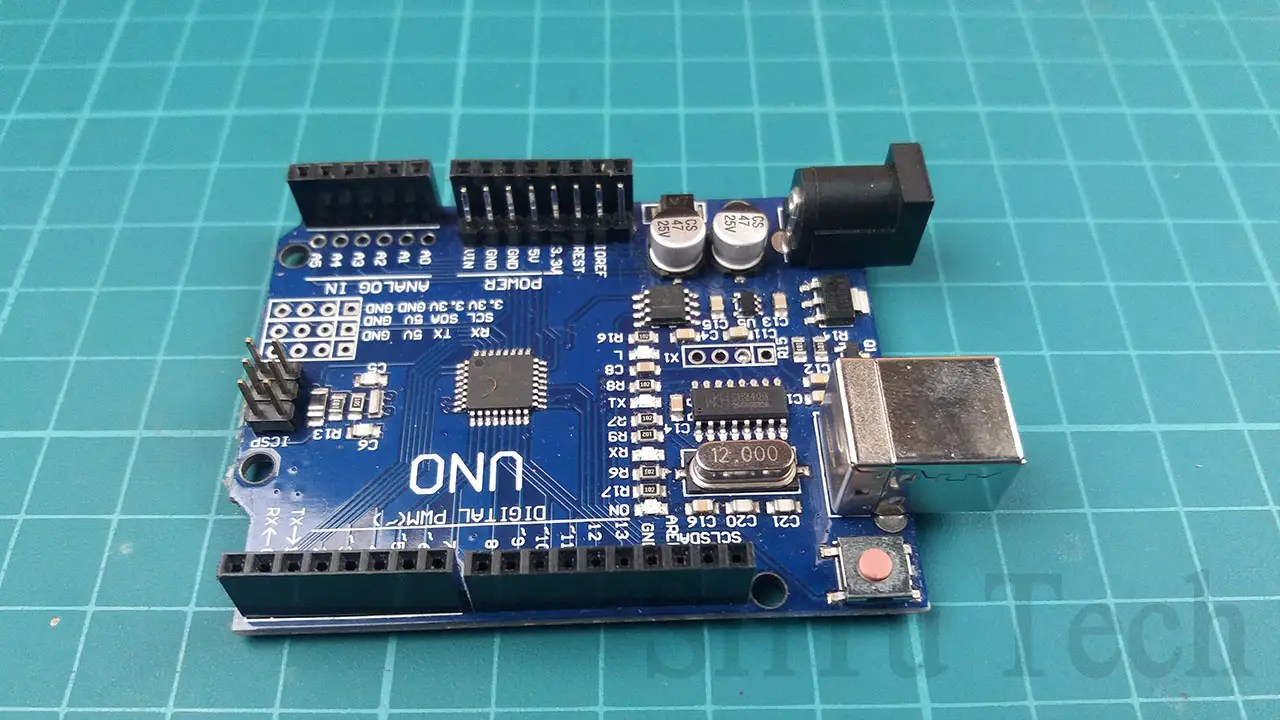You can use any other Arduino board.
Joystick module.This component is described above.
Servo motor.Below is the full description link of this component.
LED bulb220ohm and 10k resistorThe LED bulb uses a 220ohm resistor to control the current and the 10k resistor is used for the pull-up circuit.Use to mount components.
Jumper Wires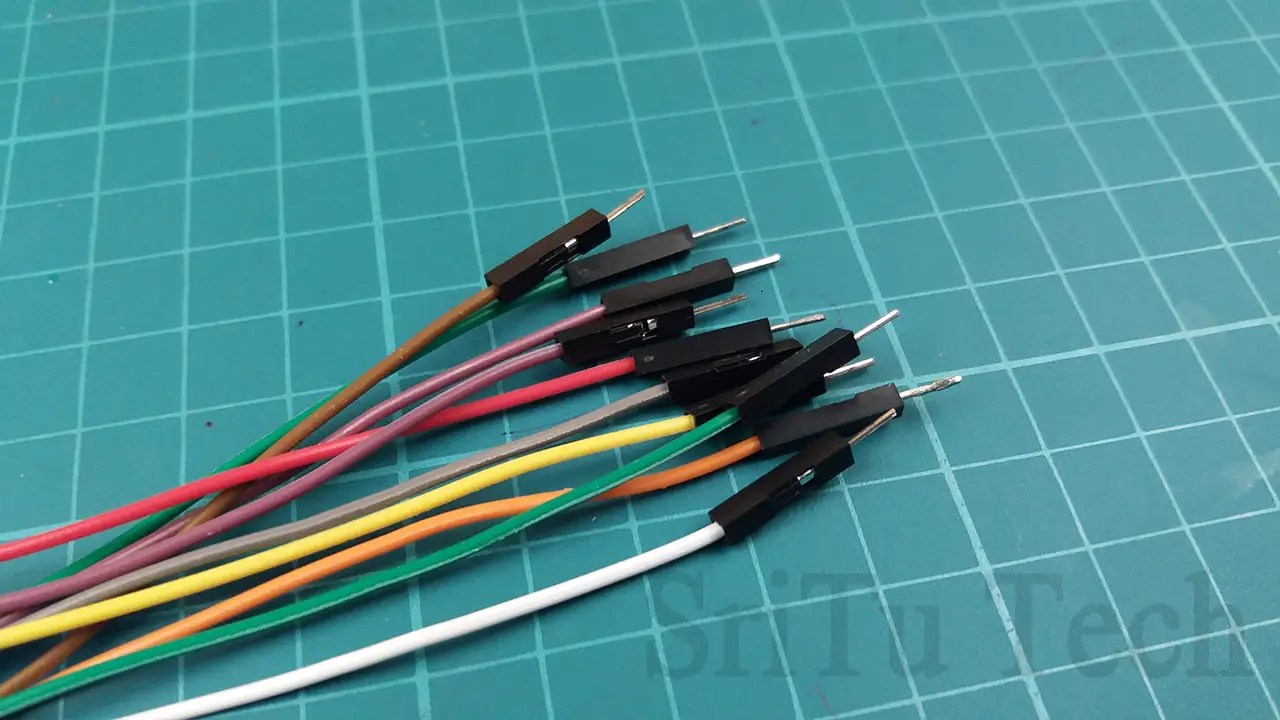Nine MALE to MALE jumper wires were used for this project.

### Step 2

Connect these components to the breadboard.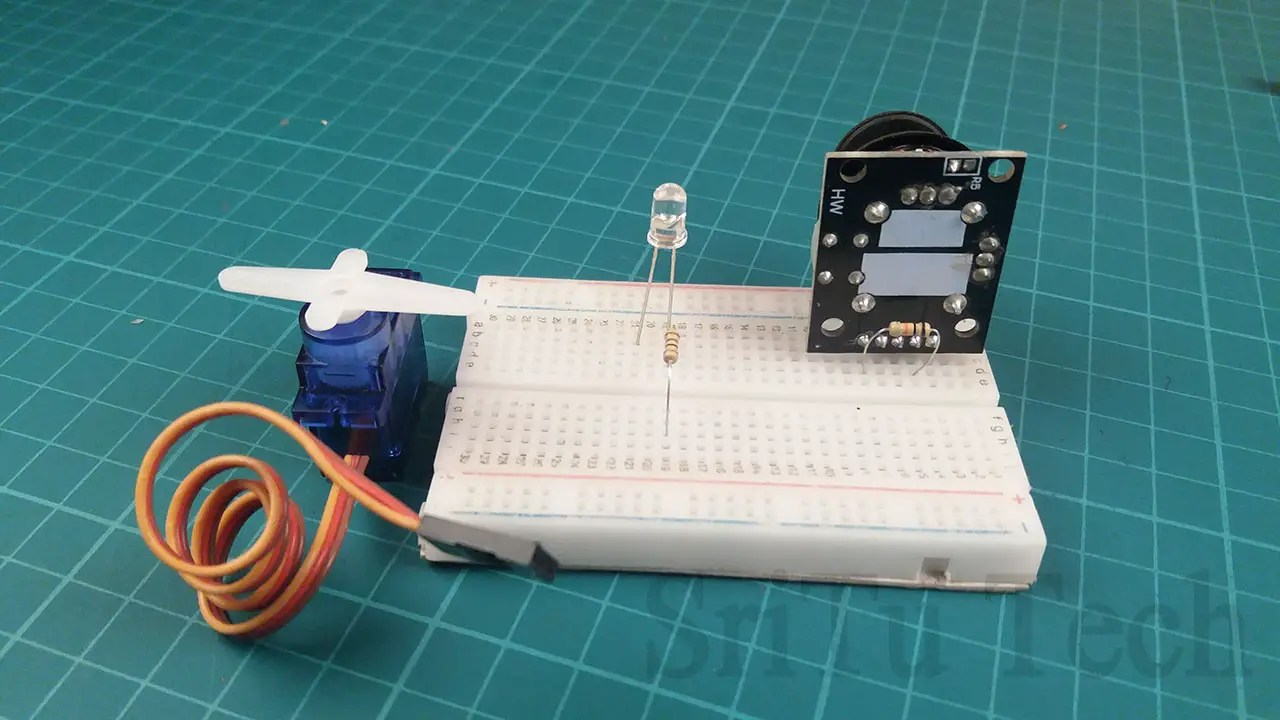### Step 3

Connect these components to your Arduino Uno board using the circuit diagram below.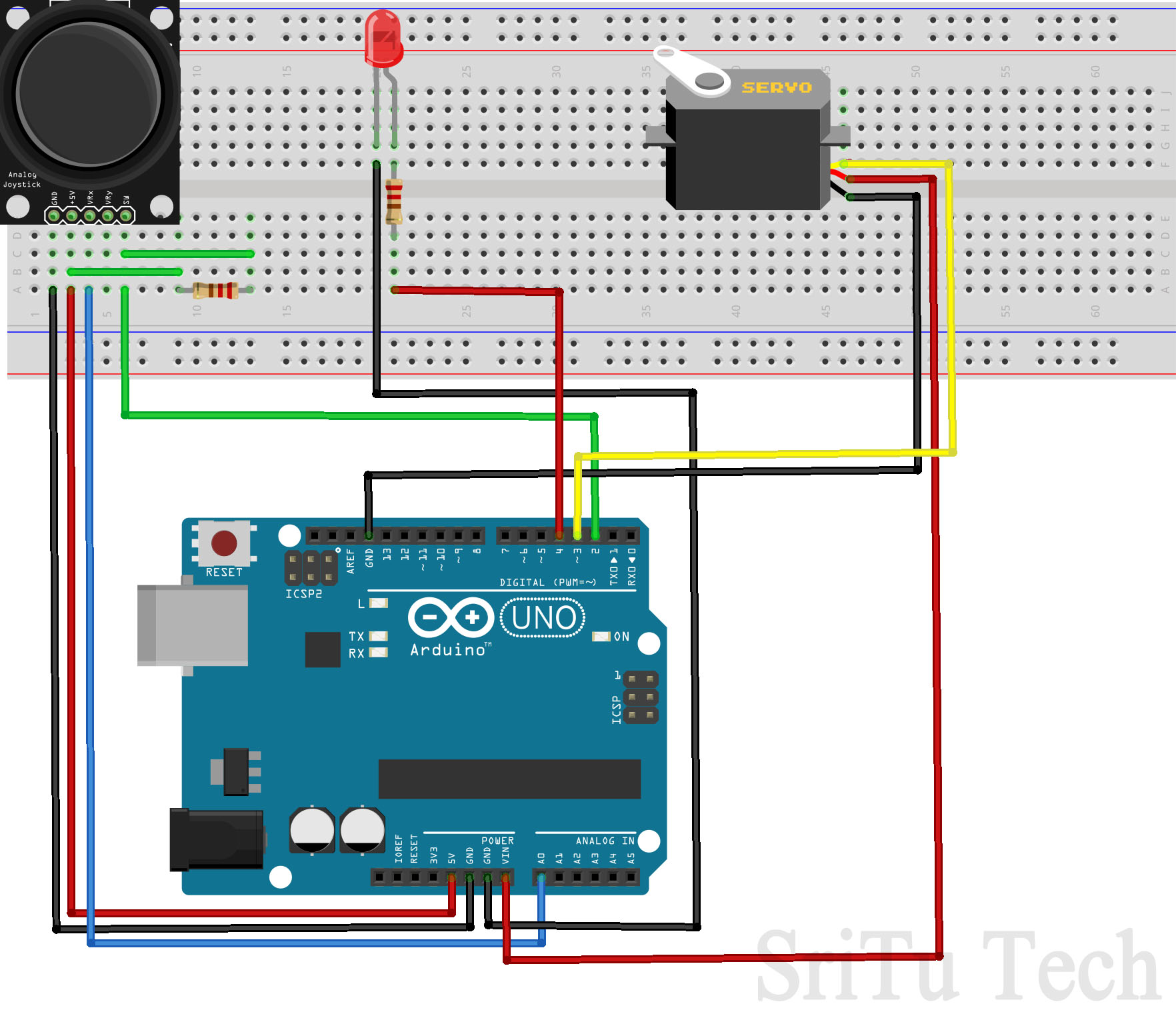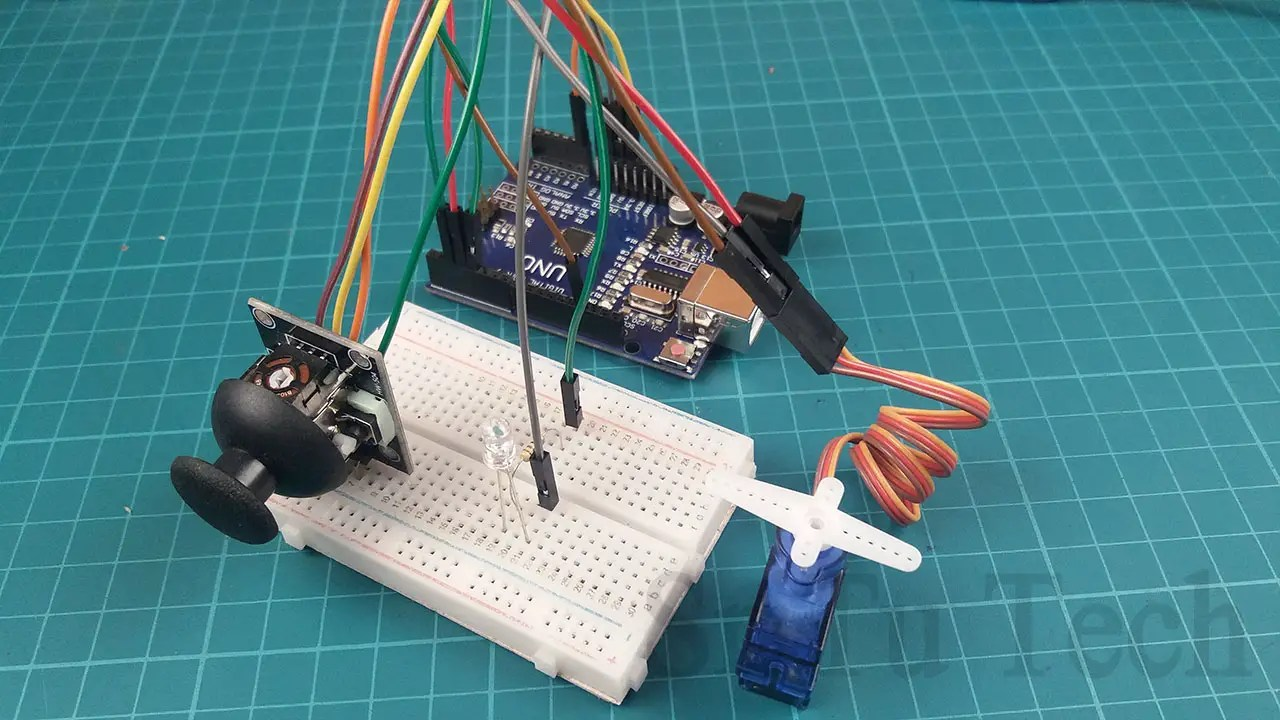Use only the PMW pins to connect the servo motor. The 220ohm resistor is used to control the current flowing through the LED bulb. The 10k resistor is used for the pull-up circuit.
Step 4
Let’s look at the code below.
``````void setup() {
pinMode(2, INPUT);
pinMode(3, OUTPUT);
pinMode(4, OUTPUT);
}
void loop() {
x = map(x, 0, 1024, 0, 180);
analogWrite(3, x);

if (b == 0) {
digitalWrite(4, HIGH);
} else {
digitalWrite(4, LOW);
}
}``````
This code sets the second pin as an input pin, the third pin as an output pin and the fourth pin as an output pin.
void setup() {
pinMode(2, INPUT);
pinMode(3, OUTPUT);
pinMode(4, OUTPUT);
}
Then this code reads analog and digital values and puts them into the variables. The integer data type named ‘x’ and boolean data type named ‘b’. This process is called variable declaring.
This code converts the analog value from 0 -1024 to 0 -180. The servo motor then rotates.
x = map(x, 0, 1024, 0, 180);
analogWrite(3, x);
The digital value is checked using an IF condition. Then it is checked whether it is equal to 0. The LED bulb turns on when the digital value is 0 and the LED bulb turns off when the digital value is 1.
if (b == 0) {
digitalWrite(4, HIGH);
} else {
digitalWrite(4, LOW);
}
Step 5
Select board and port.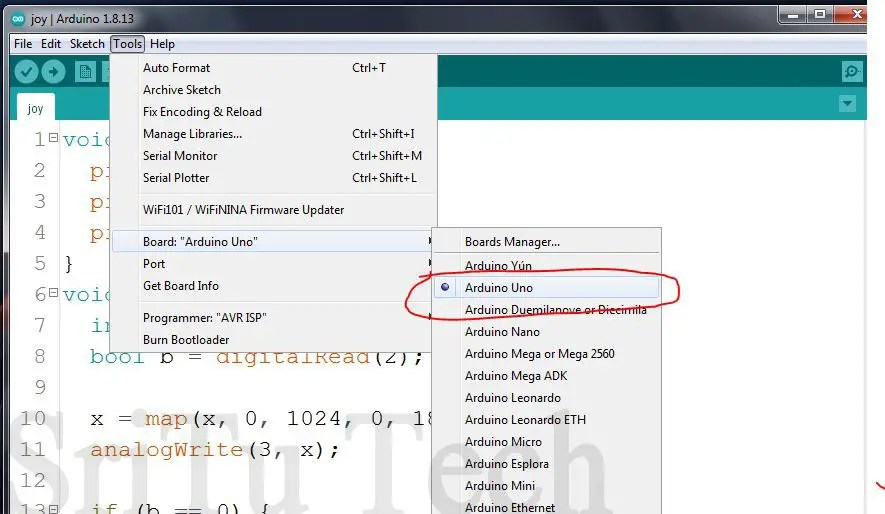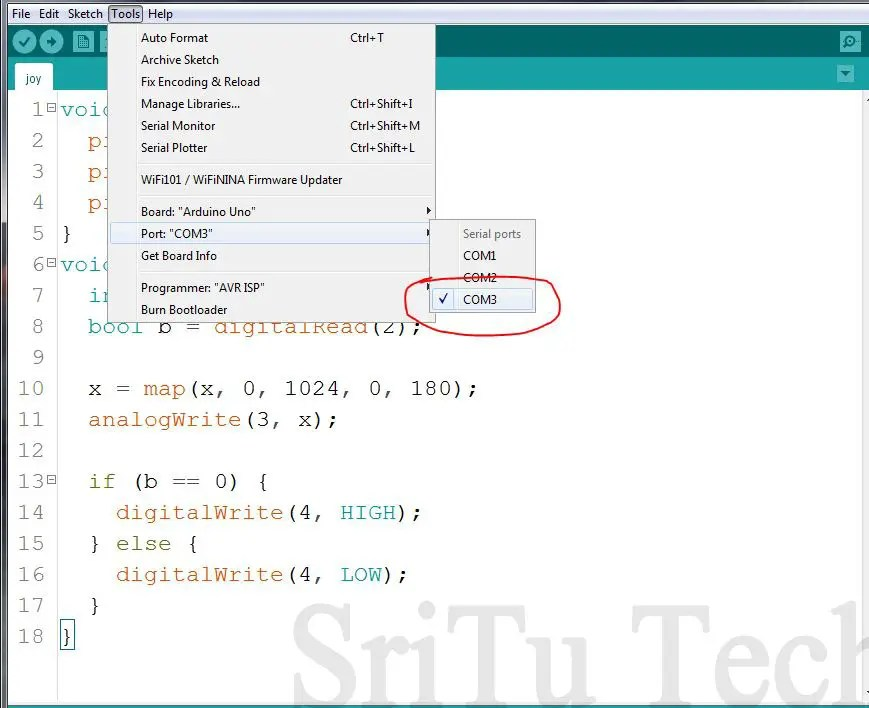Step 6
Upload code this and enjoy this video.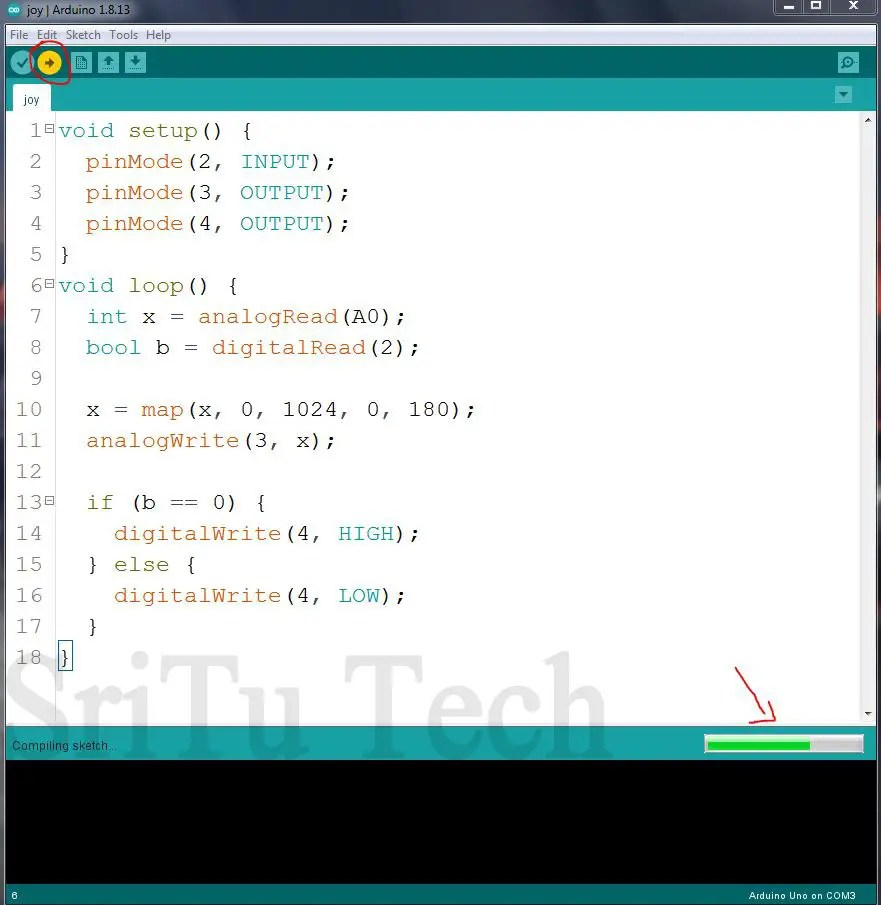Below is the video of the final results of this project. Watch it.
The following is a project using the Arduino Nano. It uses a serial monitor to view the joystick module readings. The circuit diagram and code are given below as the components and other details are described above.

### Circuit diagram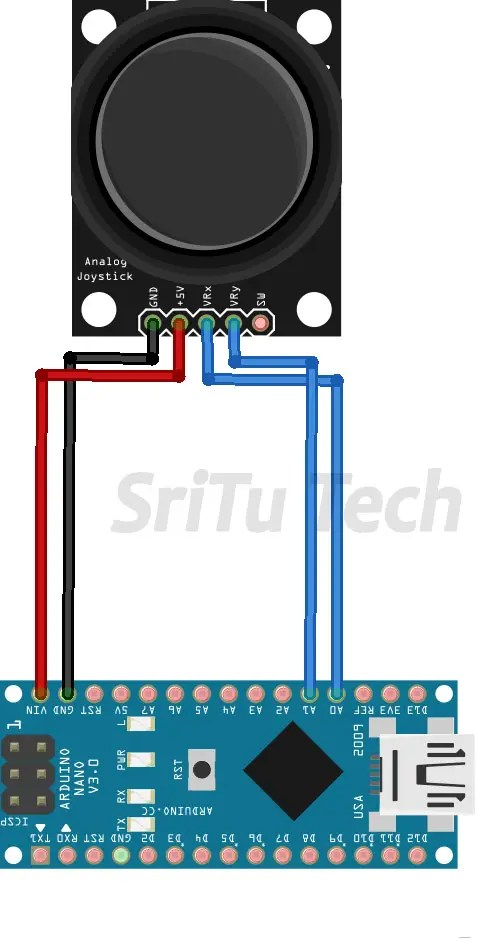### Source code

``````/*How to use the joystick module.
*created by the SriTu Tech team.
*Read the code below and use it for any of your creation
*/

void setup() {
Serial.begin(9600);//enable serial monitor
}
void loop() {
int joy = analogRead(A0);//get analog value (0-1024)
int joy1 = analogRead(A1);//get analog value (0-1024)
String x = "x axis ";//creating string variable
String y = "y axis ";//creating string variable
Serial.print(x + joy);//print x axis value
Serial.print("t");//tab space
Serial.println(y + joy1);//print y axis value
Serial.println( "");//space
delay(100);//delay
}``````

### Select board and port